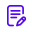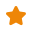# Data Science Tutorial Videos - Simplilearn

Produced in 2018

####Course Description

This Data Science Tutorial Video Playlist will help you learn what is Data Science, Data Science wth SAS, Predictive Modelling Techniques, why Data Scientist, Business Analytics, Data Science with Python, Data Science with R, Statistical Concepts, NLP, Data Visualization, PROC SQL, Data Science Algorithms etc.The topics covered in the Data Science training playlist are below: 1. Data Science basics - Introduction to Data science, predictive modelling techniques, basic analytical techniques, introduction to R, SAS, Excel, Data Science with python, data analytics overview, introduction to business analytics, linear regresion tutorial, statistical analysis tutorial, clustering tutorial, ststistical concepts, NLP, R algorithms tutorial, PROC SQL tutorial, data visualization tutorial, Data Science with R tutorial, business analytics with R tutorial etc.2. Advanced Analytics tools such as Apache Spark tutorials3. Data Collection and ampamp Storage tools such as Apache HBase tutorials 4. ETL tools such as Hive tutorial, Pig tuorial and Sqoop tutorial5. File system tools such as HDFS6. Programming tools such as Hadoop MapReduce turorial, Python tutorial and R tutorials

###Reviews

0.0

0 total reviews

5 star 4 star 3 star 2 star 1 star
% Complete
% Complete
% Complete
% Complete
% Complete